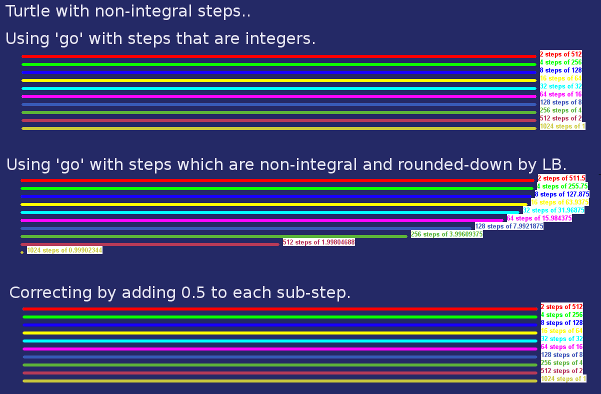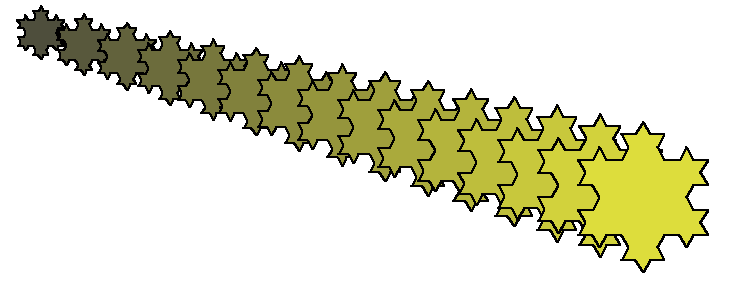# Koch using JHF's accurate turtle functions

LB's inbuilt turtle commands are great, but store turtle location and heading only as integers. As a result errors accumulate if you have a whole chain of calculated moves.By writing code to store turtle position and heading as floats, thse errors disappear.## Code

```nomainwin

WindowWidth  =800: WindowHeight =700

graphicbox #w.gb, 0,0,800,700

open "Demo Koch Snowflake" for window as #w

hw =hwnd( #w.gb)
calldll #user32, "GetDC", hw as ulong, hdc as ulong

#w    "trapclose quit"
global x, y, tx, ty, otx, oty, ta, targetcolor
tx  =0: ty  =0: ta =0   '   turtle ( x, y) and heading in degrees
otx =0: oty =0
targetcolor =1

for i =1 to 15
if targetcolor =0 then targetcolor =1 else targetcolor =0
ta =0
x =   0 + 40 *i
y =  20 + 10 *i
otx =x: oty =y
#w.gb "up ; goto "; tx; " "; ty; " ; down ; size 2"
#w.gb "color "; targetcolor; " 0 0"

l = 40 +6 *i
d =  2

for side =1 to 3
call f l, d
ta =ta +120         '#w.gb "turn 120"
next side

r =l  /150 *255'    int( 50 +200 *rnd( 1))
#w.gb "backcolor "; r; " "; r; " 60"
#w.gb "color red"

xS =x +7: yS =y +3
#w.gb "down ; size 1 ; set "; x +7; " "; y +3; " ; up ; size 2"

'goto [skip]
calldll #gdi32, "ExtFloodFill",_
hdc                as ulong,_       '   handle of graphic window
xS                 as long,_        '   x-origin of fill
yS                 as long,_        '   y-origin of fill
targetcolor        as long,_        '   boundary colour
_FLOODFILLBORDER   as long,_        '   ie fill out 'til the above colour is met...
result             as long
[skip]

next i

#w.gb "getbmp scr 1 1 800 700"
bmpsave "scr", "KochTurtle" +str\$( time\$( "seconds")) +".bmp"
wait

sub quit h\$
close #h\$
calldll #user32, "ReleaseDC", hw as ulong, hdc as ulong   'release the DC
end
end sub

sub f length, depth
scan
if depth <= 0 then
tx =otx +length *cosR( ta)
ty =oty +length *sinR( ta)      '#w.gb "go "; int( length)
#w.gb "down ; line "; otx; " "; oty; " "; tx; " "; ty; " ; up"
else
call f length /3, depth -1:     ta =ta -60'    #w.gb "turn -60"
call f length /3, depth -1:     ta =ta +120'   #w.gb "turn 120"
call f length /3, depth -1:     ta =ta -60'    #w.gb "turn -60"
call f length /3, depth -1
end if
otx =tx: oty =ty
end sub

function cosR( th)
cosR =cos( th *2 *3.14159265 /360)
end function

function sinR( th)
sinR =sin( th *2 *3.14159265 /360)
end function

```